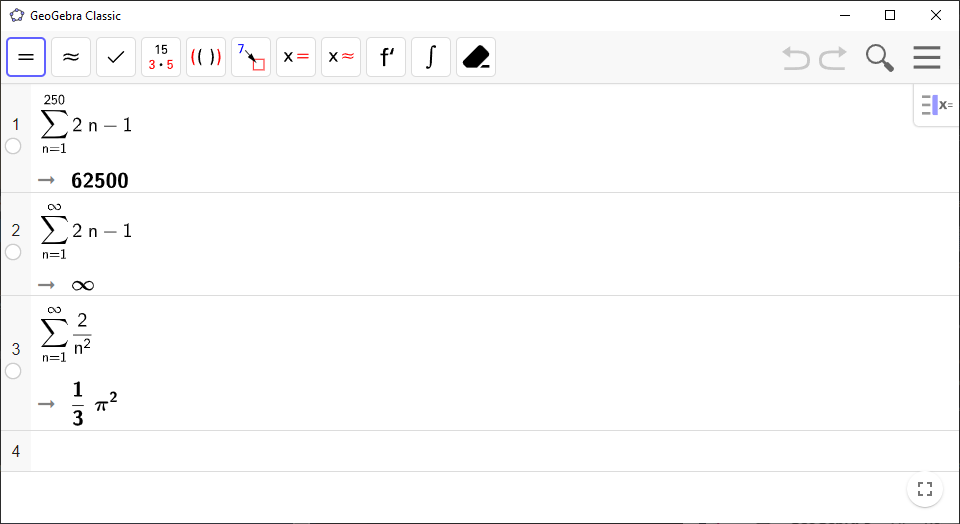# How to Use the Sum Command in GeoGebra for Sums and Series

Use `GeoGebra` to calculate sums, including infinite series.

`GeoGebra` Instruction 1

1.
Open `CAS` under`View` in`Menu`.
2.
Use the command

Sum(<Expression>, <Variable>, <Start Value>, <End Value>).

Type the expression for ${a}_{n}$ in `<Expression>`. Type $n$ for `<Variable>`. Insert the start value of $n$ in `<Start Value>`, and the end value of $n$ in `<End Value>`.

If you want to compute an infinite series, type `inf` in `<End Value>` (`inf` is short for infinity).